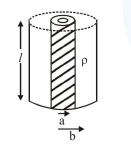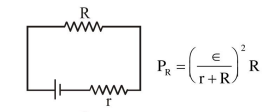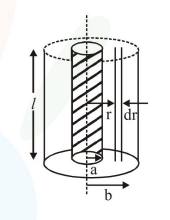# Model a torch battery of length l to be made up of a thin cylindrical bar`
Question:

Model a torch battery of length $l$ to be made up of a thin cylindrical bar of radius ' $a$ ' and a concentric thin cylindrical shell of radius 'b' filled in between with an electrolyte of resistivity $\rho$ (see figure). If the battery is connected to a resistance of value $R$, the maximum Joule heating in $\mathrm{R}$ will take place for:-1. $\mathrm{R}=\frac{2 \rho}{\pi l} l \mathrm{n}\left(\frac{\mathrm{b}}{\mathrm{a}}\right)$

2. $\mathrm{R}=\frac{\rho}{\pi l} \ln \left(\frac{\mathrm{b}}{\mathrm{a}}\right)$

3. $\mathrm{R}=\frac{\rho}{2 \pi l}\left(\frac{\mathrm{b}}{\mathrm{a}}\right)$

4. $\mathrm{R}=\frac{\rho}{2 \pi l} \ln \left(\frac{\mathrm{b}}{\mathrm{a}}\right)$

Correct Option: , 4

Solution:

Maximum power in external resistance is generated when it is equal to internal resistance of battery.$\mathrm{P}_{\mathrm{R}}$ is max. when $\mathrm{r}=\mathrm{R}$$\int \mathrm{dr}=\int_{\mathrm{a}}^{\mathrm{b}} \frac{\rho \mathrm{dr}}{2 \pi \mathrm{r} l} \Rightarrow \mathrm{r}=\frac{\rho}{2 \pi l} l \mathrm{n} \frac{\mathrm{b}}{\mathrm{a}}$